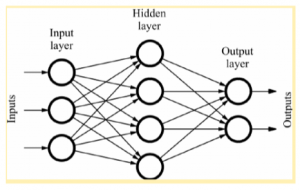# A basic introduction to Neural Networks

In the previous article – How Neural Networks can help to forecast the future – we focused on a particular usage of Neural Networks, assuming that you already had a basic knowledge concerning their structure and principles. However, it could be useful to have something we can refer to every time we talk about neural networks. That’s what you will read about in this new article.

## What are neural networks?

Maybe it would be better to say Artificial Neural Networks (ANNs) to distinguish them from Biological Neural Networks. ANNs are an attempt to model the way our brain process information: they try to simulate how neurons interact each other and transfer electric information in order to react to stimuli.

Any ANN may be divided into 3 or more layers: the input layer is a passive layer; hidden and output layers are active layers where the computation is actually performed.

Input nodes simply take the corresponding input and transfer it to the next layer nodes. In the simplest case, there is only one hidden layer that processes the information received by the previous layer and transmits the output to next nodes. The output layer outputs the results of the ANN processing.

The following figure is an example of an ANN: nodes are artificial neurons, each arrow represents the information flow that transfers the output of one neuron to the next ones.## How do single neurons exactly work?

Active neurons – neurons in active layers – are the basic computation units of each ANN. They perform a weighted sum of their inputs (each arrow has a weight that can be seen as the “strength” of the respective signal) and use an activation function to calculate the corresponding output. In the simplest case, the output could be just 0 or 1 (step activation function): is the weighted sum above a given threshold? If yes, the neuron “fires” – outputs 1 – and the information is transmitted to next neurons. Different activation functions are available, in practice.

## Where does the magic actually happen?

As you have probably noticed, changing the weights we can influence the output of single neurons and, as a result, the output of the global ANN. This can be used as a mechanism to make the ANN learn. Such a mechanism is often based on the back-propagation algorithm (at least in supervised learning). Basically, what we want is that, given an input, the ANN outputs a precise result. The general idea behind the back-propagation algorithm is to use the difference between the actual output and the desired output (the error) as a hint to adjust the weights. This learning process is repeated multiple times until we reach an acceptable output.

## How many different ANNs could we have?

The way neurons are connected determines the ANN topology: ANNs where information flows in one direction only, from input to hidden to output layers, are called feed-forward ANNs; on the other hand, feedback (or recurrent) ANNs allow signals to travel in both directions.

Anyway, given a topology, different ANNs with significantly different performances can be obtained just by changing the number of layers, nodes, connections, activation functions and other parameters. According to the application field, one ANN could be more suitable than another one, of course.

That’s all for today! I hope you enjoy the article. Keep following us and feel free to ask anything in the comments, as usual!

Graziano Mita

Data Scientist at Ezako